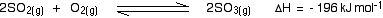Chemguide: Support for CIE A level Chemistry ``` ``` Learning outcome 7.1 Equilibria Chemical equilibria: reversible reactions, dynamic equilibrium ``` ``` ``` ``` Statement 7.1.9 This statement is about what happens to the values of equilibrium constants if you change the conditions of a reaction. This is actually very easy! With one exception, equilibrium constants are constant. Nothing happens to the value of an equilibrium constant if you change the concentrations of things taking part, or the pressure (if you are talking about gases), or if you add a catalyst. The value of an equilibrium constant stays the same - it is an equilibrium constant. The only time that an equilibrium constant will change is if you change the temperature. So learn this: Equilibrium constants are constant at constant temperature. (The implication of this is that they change if you change the temperature, but remain the same if you change anything else.) The reasons for this are covered on the page equilibrium constants and Le Chatelier's Principle. This page contains more detail than you are likely to need for CIE purposes. In particular, the maths in the section about Kp looks a bit scary - although it isn't really! Skim the page to see whether it scares you or not. If it does, ignore it! You do, however, have to be able to predict things about the effect of a change of temperature. You should already be able to work out what happens to the position of equilibrium if you increase the temperature of an equilibrium where the forward reaction is exothermic. For example:The reaction responds in such a way as to counter the effect of what you have done. That means that it would move in such a way as to absorb heat. In this case, that means in the direction of the back reaction. The position of equilibrium would move to the left. That means that there would be more reactants present at equilibrium and less product. Now, remember that equilibrium constants are always written with the products at the top and the reactants at the bottom. So if you have less product and more reactants, the value of the equilibrium constant will have decreased. If you have an equilibrium in which the forward reaction is endothermic, the reverse would happen. According to Le Chatelier, increasing the temperature would increase the amount of product, and decrease the reactants. That means that the value of the equilibrium constant would increase. Note:  I personally wouldn't try to learn this, but would work it out quickly if I needed it. You have to know about Le Chatelier's Principle, and be able to work out the effect of things like changes in temperature on positions of equilibrium. This is only a slight extension of this. All you need to remember is that in an equilibrium constant, the products are on the top and the reactants on the bottom of the expression. Make sure you understand it well enough to work it out if you need to. There is no need to learn it. You could, of course, equally well be expected to reverse this argument. You could be given equilibrium constants at two different temperatures, and be asked whether the forward reaction was exothermic or endothermic. Again, it isn't hard to work out, but potentially confusing if you try to remember it. Suppose the equilibrium constants, Kp, for a reaction were 160 at 500 K, and 54 at 700 K. Is the forward reaction exothermic or endothermic? The equilibrium constant has fallen as the temperature increased. That means that at a higher temperature there are less products and more reactants. The equilibrium has moved to the left. It moved to the left to try to counter the increase in temperature. That means that the back reaction must be endothermic, because that would absorb heat. So the forward reaction is exothermic. Please don't go on until you are sure that you can play around with these ideas with some confidence! But, above all, remember that the only thing which changes equilibrium constants is a change of temperature. ``` ``` Go to the Section 7 Menu . . . To return to the list of learning outcomes in Section 7 Go to the CIE Main Menu . . . To return to the list of all the CIE sections Go to Chemguide Main Menu . . . This will take you to the main part of Chemguide. © Jim Clark 2019